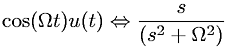Equations > Signal Processing > Laplace Transform Pairs > Laplace transform involving the unit step function and cosine

### Laplace transform involving the unit step function and cosineLatex Code:

MathML Code:

 $\mathrm{cos}\left(\Omega t\right)u\left(t\right)⇔\fracs\left({s}^{2}+{\Omega }^{2}\right)$

MathType 5.0: